# VBA colors

## 0. Quick guide to the RGB color model

In this module:

1. RGB color circles
2. ColorIndex property - 56 colours, and VBA ColorConstants
3. RGB and decimal color values, plus conversion
4. Color property

The RGB color model adds combinations of Red, Green, and Blue to produce various colours. Each component is an integer value 0 to 255. The RGB colors with intersection overlap is demonstrated in figure 1.

## 1. RGB colors

### 1.1 RGB circles with additive colour mixing

Three RGB circles with colour mixing - returns 7 colors.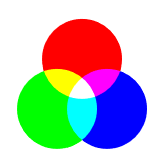Fig 1: - three circles; Red, Green, and Blue (RGB), with 4 intersection points

With the inclusion of black (no colour), the eight colours are:

1. Black: RGB(0,0,0)
2. White: RGB(255,255,255)

3. Red: RGB(255,0,0)
4. Green: RGB(0,255,0)
5. Blue: RGB(0,0,255)

6. Yellow: RGB(255,255,0)
7. Magenta: RGB(255,0,255)
8. Cyan: RGB(0,255,255)

### 1.2 RGB circles - production code

Using web browser CSS and Scalable Vector Graphics (SVG)

Code for CSS style and Scalable Vector Graphics (SVG) used to produce the RGB circles in figure 1

circle {mix-blend-mode: screen;}



## 2. VBA ColorIndex property

Syntax: expression.ColorIndex = value

where value is an element from the integer series 1,2, …, 56. Special values include xlColorIndexAutomatic (-4105) and xlColorIndexNone (-4142).

Examples of the ColorIndex property:

• xlRange.Value = "excel"
• xlRange.Interior.ColorIndex = 48
• xlRange.Font.ColorIndex = 20
• xlRange.Borders.ColorIndex = 3
• xlRange.Characters(1, 2).Font.ColorIndex = 6

### 2.1 The 56 colours of ColorIndex

Colours 2 to 8 are red, green, and blue with additive mixing. The colours 9 to 56 are various combinations of red, green and blue with RGB values: 0, 51, 102, 128, 150, 153, 192, 204, and 255 (figure 2).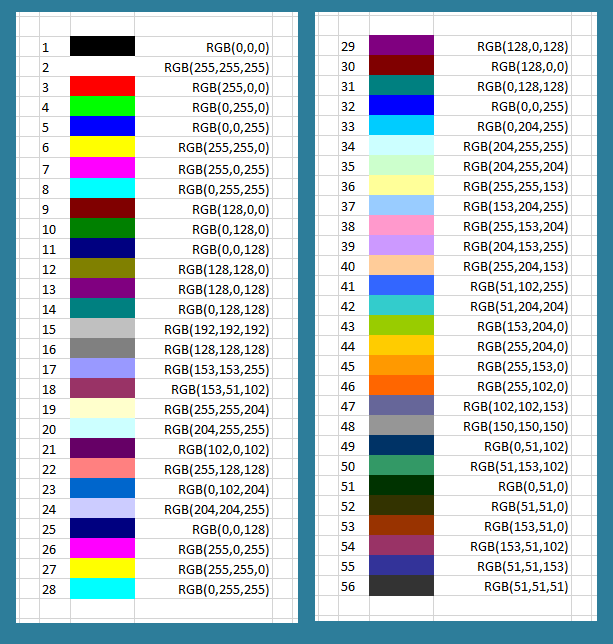Fig 2: ColorIndex - numbers, colours, and RGB values from code 1

The WS range in figure 2 was printed from the ColIndx2wWS procedure in code 1.

Code 1: Sub ColIndx2WS procedure prints ColorIndex to WS (see figure 2)
Sub ColIndx2WS()
' Requires xlfDec2RGB(ColDec) function
Dim i As Integer
Dim AC As Range: Set AC = ActiveCell

For i = 1 To 56
AC(i, 1) = i
AC(i, 1).HorizontalAlignment = xlLeft
AC(i, 2).Interior.ColorIndex = i
AC(i, 3).Value = "RGB(" & xlfDec2RGB(AC(i, 2).Interior.Color) & ")"     ' see code ...
AC(i, 3).HorizontalAlignment = xlRight
Next i
AC(i, 3).ColumnWidth = 19
End Sub



The colour table can also be printed as a 7 by 8 array (code 2)

Code 2: Sub ColIndx2WSarraS procedure prints ColorIndex to WS array (7 rows by 8 columns)
Sub ColIndx2WSarray()
Const TLC As String = "B2"      ' top left cell
Dim i As Integer, j As Integer
Dim Col As Integer, Val As Integer

With Range(TLC)
For i = 1 To 7
For j = 1 To 8
Col = i * j
Val = Val + 1
.Offset(i - 1, j - 1).Interior.ColorIndex = Col
.Offset(i - 1, j - 1).Value = Val
Select Case Col
Case 1, 5, 9 To 14, 16, 18, 21, 23, 25, 29, 30, 32, 41, 47 To 49, 51 To 56
.Offset(i - 1, j - 1).Font.ColorIndex = 2
Case Else
.Offset(i - 1, j - 1).Font.ColorIndex = 1
End Select
Next j
Next i
.Resize(7, 8).ColumnWidth = 5
.Resize(7, 8).RowHeight = 20
End With

End Sub



Ten of the ColorIndex colours are duplicate pairs: Blue    5    and    32   ; Yellow    6    and    27   ; Pink    7    and    26   ; Turquoise    8    and    28   ; Dark Red    9    and    30   ; Dark Blue    11    and    25   ; Violet    13    and    29   ; Teal    14    and    31   ; [No name]    18    and    54   ; and [No name]    20    and    34    . Leaving only 46 unique colours.

### 2.2 ColorConstants

The 8 colours listed in section 1.1 have name equivalents listed as members of the VBA ColorConstants class in the decimal colour system. The ColorConstants Auto List drop down is shown in figure 4.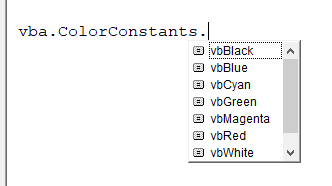Fig 4: VBA ColorConstants - VBA Auto List drop down with 8 items

Code 3 prints a list of the ColorConstants numerical values to the immediate window (figure 5).

Code 3: Sub ColConst procedure
Sub ColConst()
Dim i As Integer
Dim ColArrVal As Variant
Dim ColArrLbl As Variant

ColArrVal = Array(vbBlack, vbWhite, vbRed, vbGreen, vbBlue, vbYellow, vbMagenta, vbCyan)
ColArrLbl = Array("vbBlack", "vbWhite", "vbRed", "vbGreen", "vbBlue", "vbYellow", "vbMagenta", "vbCyan")

For i = LBound(ColArrVal) To UBound(ColArrVal)
Debug.Print ColArrLbl(i) & ": " & ColArrVal(i)
Next i

End Sub

The immediate window with output from line 69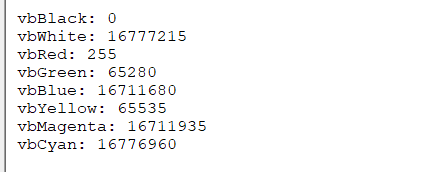Fig 5: VBA ColorConstants - colors 1 to 8

## 3. Decimal values of colours

### 3.1 RGB to positive decimal

To convert RGB to decimal , the relationship is $C_d = R * 256 ^ 0 + (G * 256 ^ 1) + (B * 256 ^ 2)$. See code 4.

Code 4: Function xlfRGB2DecX converts RGB values to decimal. Includes test routine
Function xlfRGB2DecX(Red As Integer, Green As Integer, Blue As Integer) As Long
xlfRGB2DecX = Red * 256 ^ 0 + Green * 256 ^ 1 + Blue * 256 ^ 2
End Function

' ===========================
Function xlfRGB2DecY(Red As Integer, Green As Integer, Blue As Integer) As Long
xlfRGB2DecY = RGB(Red, Green, Blue)
End Function

' ===========================
Sub TestxlfRGB2DecX()
Dim Ans As Long
Ans = xlfRGB2DecX(204, 255, 255)
End Sub



### 3.2 RGB to negative decimal

To convert RGB to Excel negative decimal, the relationship is $C_{-d} = RGB - (256^3) - 1$

The color red is RGB(255,0,0), equal to 256 as a positive value. The Excel negative value equivalent is RGB - (256^3) - 1 = 256 - (256^3) - 1 = -16776961

### 3.3 Decimal to RGB

The backslash operator "\" divides two numbers and returns the integer quotient. The remainder is ignored.

Code 5: Function xlfDec2RGB converts color decimal to RGB comma separated values. Includes test routine
Function xlfDec2RGB(ByVal ColDec As Long) As String
Dim R As Long
Dim G As Long
Dim b As Long

R = (ColDec And &HFF) \ 256 ^ 0      ' &HFF hexadecimal = 255 decimal
G = (ColDec And &HFF00&) \ 256 ^ 1   ' &HFF00& hexadecimal = 65280 decimal
' trailing & is a TDC for type long, if
' omitted (&HFF00), the assigned value is -256
b = ColDec \ 256 ^ 2
xlfDec2RGB = CStr(R) & "," & CStr(G) & "," & CStr(b)

End Function

' ===========================
Sub TestxlfDec2RGB()
Dim Ans As String
Ans = xlfDec2RGB(16737843)  ' returns 51,102,255
Stop
End Sub



• Line 95: 16737843 And 255 returns 51, 51 \ 1 returns 51, remainder 0
Further details of the binary And are provided in figure 5. Only the last 8 digits on the right are relevant.
• 16737843 = 111111110110011000110011;
• 255 = 11111111;
• AND returns 00110011 = 51
•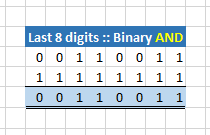Fig 5: binary AND - last 8 digits on the right
• Line 97: 16737843 And 65280 returns 26112, 26112 \ 256 returns 102, remainder 0
• Line 100: 16737843 \ 65536 returns 255, remainder 2616

## 4. Color property

Syntax: expression.Color = value

where value can be created by the RGB function returned as a long whole number.

Examples of the Color property:

• xlRange.Value = "excel"
• xlRange.Interior.Color = RGB(150,150,150)  (equivalent to ColorIndex 48)
• xlRange.Font.Color = 16777164  (equivalent to: RGB(204,255,255); ColorIndex 48)
• xlRange.Borders.Color = RGB(150,0,0)  (equivalent to ColorIndex 3)
• xlRange.Characters(1, 2).Font.Color = 6  (equivalent to: RGB(6,0,0))

• RGB discussion [23 Apr 2018]

• Development platform: Excel 2016 64 bit.
• Published: 14th April 2016
• Revised: Friday 24th of February 2023 - 10:37 PM, Pacific Time (PT)